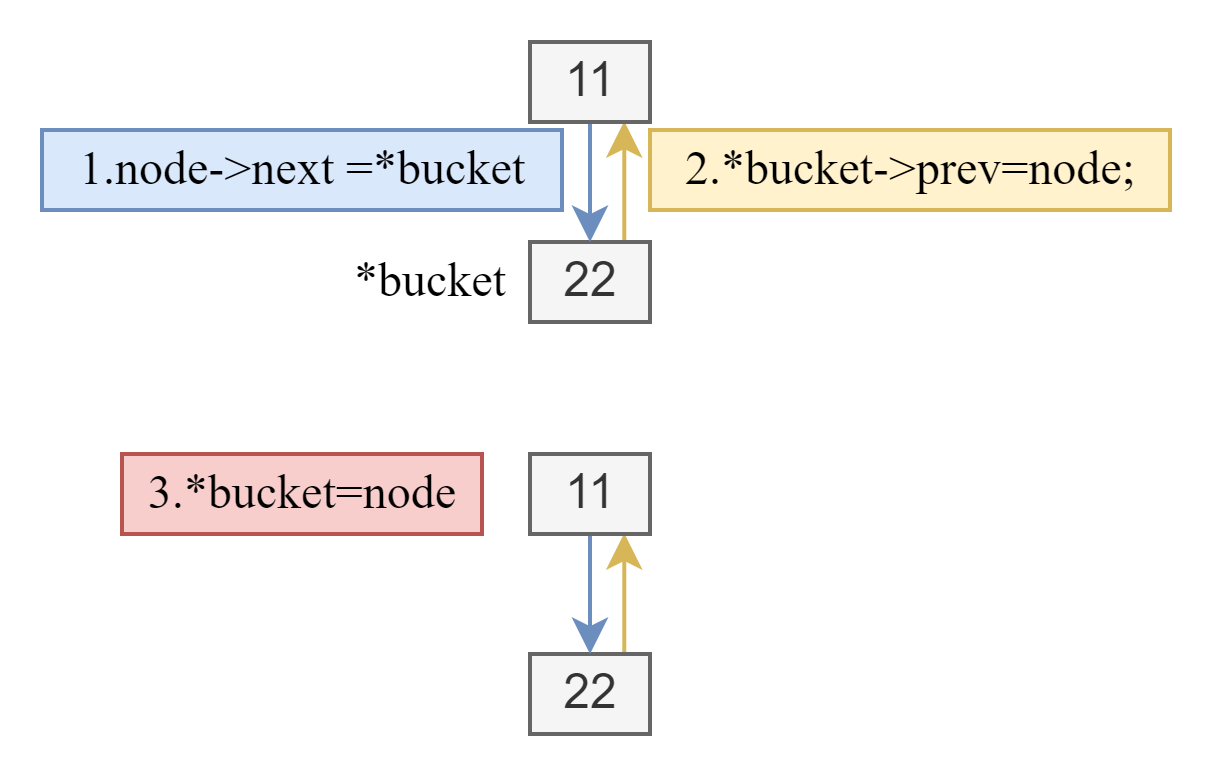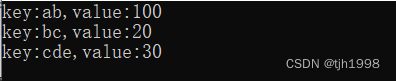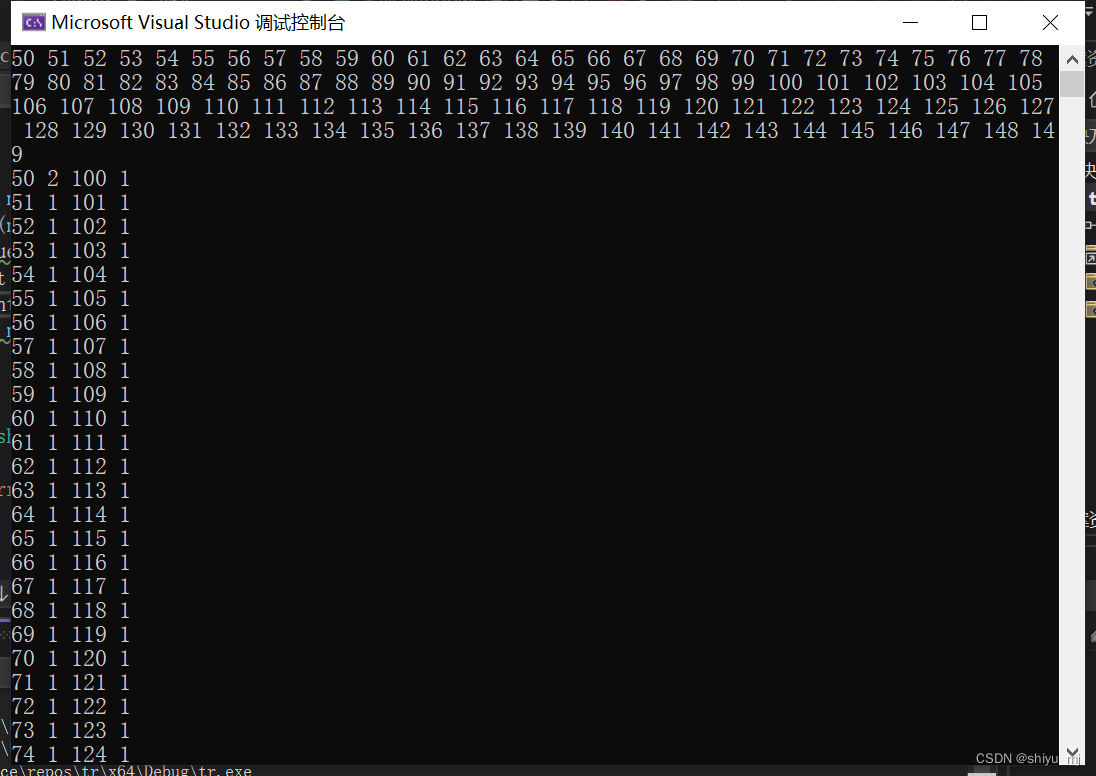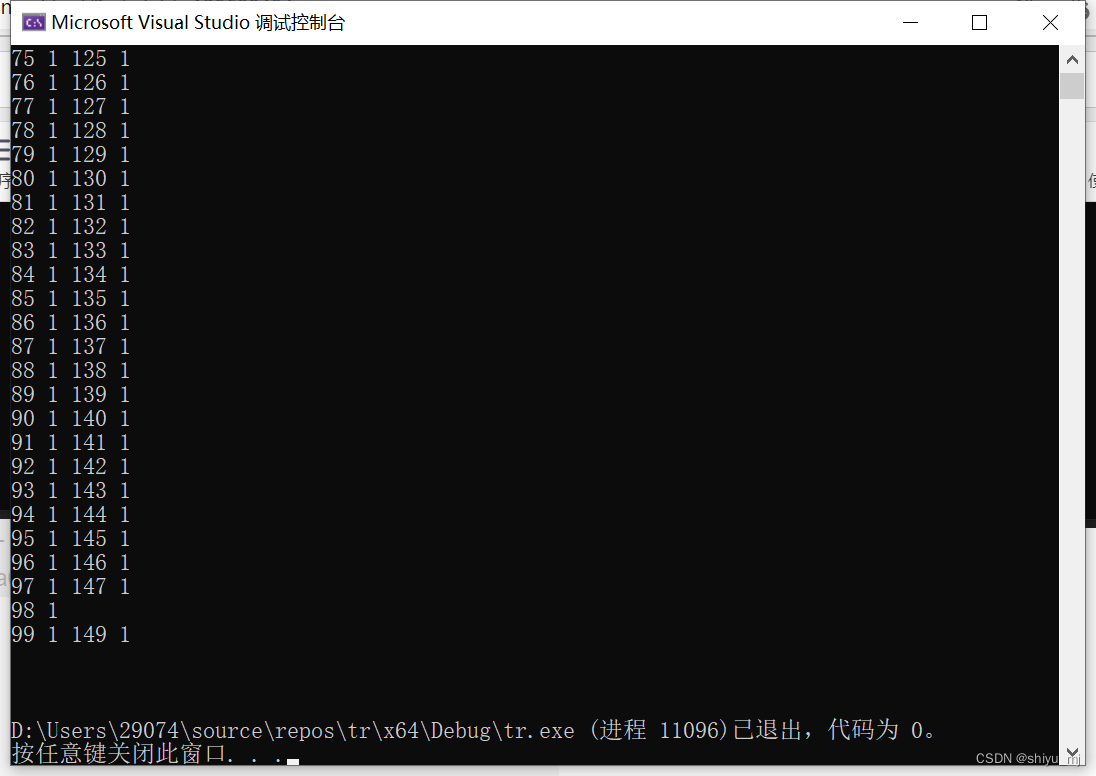• c语言实现hash表
2021-12-29 22:52:54

# 实现hash表

## 一、如何理解hash？

1. C语言中，如果数据被存放到数组中 。可以使用 arr 这种方法直接访问arr数组的第四个元素。

2. 这是一种非常快的访问方法。使用下标访问。

3. 把数组理解成hash表，而这个3 理解成key值。那么可以得到：

int hash_arr_get_value(int *arr,int key)
{
return arr[key];
}

4. 那如果这个key值不是一个数字呢？比如说是一个字符串。我需要通过一个字符串，得到对应的值。比如，我需要通过名字得到手机号码:

char *hash_arr_get_number(char **arr,char *str_key)
{
/*需要先把number转成一个key值。*/
unsigned int key = hash(str_key);
/*然后访问真正的数据*/
return arr[key];
}

5. 4中的 arr，就是一个hash表。而把key从别的数据转成一个整数下标的函数，就是hash函数。

6. 使用这种hash表存储，当要查询数据的时候，不用每次遍历查找，只需要通过固定计算，使用下标查找到想要的结果。算法时间复杂度为O(1).

## 二、使用C语言实现一个hash

1. 具体代码参考这里：hash表实现;

2. 简单的讲解：

1. 我写的这个hash依赖了 value 的模块。这是为了方便管理hash表的key和值。如果写死了key是string,value也是string，后续的扩展就变得十分困难。

/*
将value抽象一下，以后不管是字符串，还是函数指针，还是别的什么值，都可以通过修改抽象的成员解决。
*/
typedef int (*on_destory_cb)(void *);
typedef struct value_t
{
void *p;
int size;
on_destory_cb on_destroy;
}value_t;


2. value_create 和 value_destroy是对应的。如果创建了，就要销毁。因为C语言需要考虑分配空间和释放空间。

3. hash表操作函数。hash表也是一种存储方式。也是需要增删改查的操作。

/**
* 创建一个hash表。输入 表的大小和hash函数。对于不同的场景，使用不同的hash函数。
*/
fv_hash_t *hash_create(int size,hash_calculate_cb hash_fn,hash_cmp_cb hash_cmp);

/**
* 向hash表中添加元素。如果key值已经存在，会修改原来的元素。
*/
int hash_update(fv_hash_t *hash,value_t *key,value_t *data);

/**
* 从hash表中删除key值对应的元素。如果元素不存在，返回-1.删除失败返回-1.
*/
int hash_remove(fv_hash_t * hash,value_t *key);

/**
* 获取hash表中key值对应的data数据的指针。
*/
value_t *hash_get(fv_hash_t *hash,value_t *key);

/**
* 遍历hash表中的元素。key值和data的值。还有参数。
*/
int hash_foreach(fv_hash_t *hash,hash_visit_cb on_visit,void *param);

/**
* 计算hash表中有多少元素
*/
int hash_get_size(fv_hash_t *hash);

/**
* 销毁整个hash表。
*/
int hash_destory(fv_hash_t *hash);


## 三、如何解决hash冲突

1. 使用外部拉链法解决hash冲突。也就是，如果新插入一个数据到A槽，A槽已经被占用了。怎么办？就弄个链表，把新的数据插入到原来数据所在的来链表中。

2. 简单看看hash_update函数

/**
* 向hash表中添加元素。如果key值已经存在，会修改原来的元素。
* 如果key值已经存在，就是用头插法，插到最前面。这样用户检查的时候永远得到的是最前面的数据。
* 这里的实现需要完善。因为如果用户一直插一样的值，会浪费内存。我不想加删除的代码，是因为担心一直循环查找会不会降低性能。
*/
int hash_update(fv_hash_t *hash,value_t *key,value_t *data)
{
return_value_if_fail(hash != NULL && key != NULL && data != NULL,-1);
fv_hash_elem_t *hash_elem = NULL;
/*hash函数非空*/
if(hash->hash_fn)
{
/*计算key值*/
unsigned int key_index = hash->hash_fn(key->p)%hash->capacity;
/*创建 fv_hash_elem_t 对象。*/
hash_elem = hash_elem_create(key,data);
return_value_if_fail(hash_elem,-1);
/*如果当前hash槽已经被占用*/
if(hash->table[key_index] != NULL)
{
hash_elem->next = hash->table[key_index];
hash->table[key_index] = hash_elem;
}
else
{
hash->table[key_index] = hash_elem;
}
hash->size++;
}

return 0;
}


## 四、如何保证hash的性能

1. 创建大小合理的散列表。

1. 负载 因子：是当前插入到表的元素个数和可用槽的总数的比，得到的结果。

a = n/table_size

2. 如果负载因子变大，性能会下降。如果小于0.2，再让它变小，对于性能的影响特别小。

3. 我觉得可能0.5-1会好些吧。

2. 确保散列表的槽数是一个素数。

3. 需要使用代表性数据测试。最好只使用一个除法运算，就是最后n%table_size的模运算。

4. 考虑冲突，在可能的地方使用外部拉链法。

## 五、使用

1. 参考 示例代码
2. 使用场景：
1. 频繁的查找，而且对性能有要求的地方。需要使用hash表。
更多相关内容
• ## c语言实现哈希表

千次阅读 2022-03-23 21:00:27
哈希

最近在做FTP服务器项目的时候，需要统计服务器的最大连接数和每IP连接数。
每IP连接数：指的是服务器连接同一个IP地址的次数
最大连接数：服务器最多可以连接客户端的个数
其中前者需要用到哈希表。
c++中，我们可以直接使用map或者unordered_map来产生pair键值对，c语言中没有对应的库，所以需要自己实现一下hash表的结构。
如下图所示：

1. hash表中保存的是哈希函数，桶结点的个数，以及二级指针：存储的是桶结点的地址。
2. 每一个桶结点中存放的是哈希结点的地址，是一个一级指针，桶结点的个数为4个。
3. 每一个hash结点是由key指针，value指针，结点的前驱指针，后继指针构成的。这里之所以存放的是指针，是因为它可以接收任意类型的数据。

一般哈希函数选择的是除留余数法
hash(key)=key%p,其中p≤buckets，取最接近于buckets的质数即可。
需要注意的是，由于经过哈希函数映射之后，关键码对应的位置对应滴位置可能是相同的，因此采用开散列的方法，使用链表的结构将所有相同位置的关键码串联起来。
如下图所示：为了方便起见，11，22，33处对应的是哈希结点的地址而不是key值。链表的插入采用的是头插，提升效率。
例如key=0,11,22,33对应的地址都是相同的，可以采用链表的方式将其连接。1.hash_node的结构：

typedef struct hash_node
{
void *key;
void *node;
struct hash_node* prev;
struct hash_node* next;
}hash_node_t;


2.hash表的结构
//函数指针 对应的函数名是hashfunc_t:参数一个为int，一个为void。经过typedef之后，可以通过hashfunc_t来代表函数指针。

typedef unsigned int (*hashfunc_t)(unsigned int, void*);


struct hash
{
unsigned int buckets; //对应的桶的大小
hashfunc_t hash_func; //保存的hash函数的地址
hash_node_t **nodes; //二级指针，存放桶结点的首地址
};


3.hash表的生成
类型的重定义

typedef struct hash hash_t; 将hash表重定义为hash_t


# 哈希表的初始化

hash_func是自己定义的，这里存放的是函数地址。定义的hash_func如下所示：

unsigned int hash_func(unsigned int bucket_size, void* key)
{
return (*(unsigned int*)key) % bucket_size;
}


注意，这里结点的大小是桶结点的个数乘以每一个指针的内存大小，对hash_nodes所指的内存空间进行内存的初始化，指针置为NULL。

hash_t *hash_alloc(unsigned int buckets, hashfunc_t hash_func)
{
hash_t *hash = (hash_t *)malloc(sizeof(hash_t));
assert(hash != NULL);
hash->buckets = buckets;
hash->hash_func = hash_func;
int size = buckets * sizeof(hash_node_t *);
hash->nodes = (hash_node_t **)malloc(size);
memset(hash->nodes, 0, size);
return hash;
}


下面部分是获取关键码对应的桶结点的位置，通过hash函数映射之后计算出对应的bucket，将对应结点的地址返回。

hash_node_t** hash_get_bucket(hash_t* hash, void* key)
{
unsigned int bucket = hash->hash_func(hash->buckets, key);
if (bucket >= hash->buckets)
{
fprintf(stderr, "bad bucket lookup\n");
exit(1);
}
return &(hash->nodes[bucket]);
}


下面部分是通过关键值key来查询对应的结点，例如我们需要查找key=22的结点，找到buckets的位置之后，链表向后遍历即可。
之前自己理解的时侯，总觉得桶结点已经找到了，为什么还需要向后遍历呢，其实是需要的，因为当前桶的头结点存储的key值可能不是我们需要找的，比如上面的例子中，buckets存储的是key=0的元素的地址。

1. 获取当前key值对应的桶结点的地址。
2. node指向当前的桶结点的头部，依次向后寻找，直到node指向的key值和要寻找的key值相等时，代表找到了对应的结点，因此返回该结点即可。
3. memcmp（void * str1,void * str2,size_t n）用来对比str1和str2前n个字节大小是否相等。
hash_node_t* hash_get_node_by_key(hash_t* hash, void* key, unsigned int key_size)
{
hash_node_t** bucket = hash_get_bucket(hash, key);
hash_node_t* node = *bucket;
if (node == NULL)
{
return NULL;
}
while (node != NULL && memcmp(node->key, key, key_size) != 0)
{
node = node->next;
}
return node;
}


# 查询key值对应的value值

代码如下所示：如果找到key值，就返回当前key值对应的value值。否则返回NULL

void* hash_lookup_entry(hash_t* hash, void* key, unsigned int key_size)
{
hash_node_t* node = hash_get_node_by_key(hash, key, key_size);
if (node == NULL)
{
return NULL;
}
return node->value;
}


# 向哈希表中添加结点

需要给定key值的地址，对应的大小，value值的地址，对应的大小。

1. 首先查找，如果已经存在相同的key值，那么程序就会报错。
2. 其次申请新的hash结点，对前驱和后继赋空。将key和value的值拷贝到node->key和node->value中。
3. 获取当前key值对应的bucket的地址。
4. 如果为空，代表不存在，直接将结点插入，如果不为空，代表地址发生了冲突，因此需要将新的结点插入到头部。void hash_add_entry(hash_t* hash, void* key, unsigned int key_size, void* value, unsigned int value_size)
{
if (hash_lookup_entry(hash, key, key_size))
{
fprintf(stderr, "duplicate hash key\n");
return;
}
hash_node_t* node = malloc(sizeof(hash_node_t));
node->prev = NULL;
node->next = NULL;
node->key = malloc(key_size);
memcpy(node->key, key, key_size);
node->value = malloc(value_size);
memcpy(node->value, value, value_size);
hash_node_t** bucket = hash_get_bucket(hash, key);
if (*bucket == NULL)
{
*bucket = node;
}
else
{
// 将新结点插入到链表头部
// 当前结点的后继指向下一个结点。
node->next = *bucket;
//
(*bucket)->prev = node;
*bucket = node;
}
}


# 释放hash表中的结点

1. 检查key值是否存在，存在的话，释放对应的key和value。
2. 如果被释放的结点存在前驱指针，那么前驱指针的下一个指针指向当前被释放结点的下一个结点。如果不存在前驱指针，那么说明当前指针是头结点，故找到对应的桶结点的位置，将桶结点指向当前结点的下一个结点。
3. 当前结点下一个结点不为空，下一个结点的前驱结点指向当前结点的前驱结点。void hash_free_entry(hash_t* hash, void* key, unsigned int key_size)
{
hash_node_t* node = hash_get_node_by_key(hash, key, key_size);
if (node == NULL)
{
return;
}
free(node->key);
free(node->value);
if (node->prev)
{
// 第一步
node->prev->next = node->next;
}
else
{
hash_node_t** bucket = hash_get_bucket(hash, key);
*bucket = node->next;
}
// 后继结点的前驱连接被删除结点的前驱（第二步）
if (node->next)
node->next->prev = node->prev;
// 释放结点
free(node);
}


附录：假设给定的key值不是int值，要怎么处理呢？参考了一下别人的代码：将对应的字符串转为相对应的ASII值，再进行计算即可。

unsigned int hash_func_str(unsigned int bucket_size, char* key)
{
int sum = 0;
char* tmp = key;
while (*tmp != '\0')
{
sum += *tmp;
tmp++;
}
return sum % bucket_size;
}


# 打印hash表

1.key值为数字的时候

void printhash(hash_t* hash1)
{
hash_node_t** z = hash1->nodes;
int i = 0;
for (i = 0; i < hash1->buckets; ++i)
{
if (z[i] == NULL)
continue;
while (z[i]!= NULL)
{
printf("key:%d,value:%d\n", *(int*)(z[i]->key), *(int*)(z[i]->value));
z[i] = z[i]->next;
}
}
}


2.key值为字符串的时候

void printhash(hash_t* hash1)
{
hash_node_t** z = hash1->nodes;
int i = 0;
for (i = 0; i < hash1->buckets; ++i)
{
if (z[i] == NULL)
continue;
while (z[i]!= NULL)
{
printf("key:%s,value:%d\n", (char*)z[i]->key, *(int*)(z[i]->value));
z[i] = z[i]->next;
}
}
}


测试函数如下所示：

static struct hash* ip_count_hash;
int main()
{
ip_count_hash = hash_alloc(17, hash_func_str);
char key1[] = "ab";
//printf("%d\n", sizeof(key1));
int value1 = 100;
char key2[] = "bc";
int value2 = 20;
char key3[] = "cde";
int value3 = 30;
hash_add_entry(ip_count_hash, &key1, sizeof(key1), &value1,sizeof(int));
hash_add_entry(ip_count_hash, &key2, sizeof(key2), &value2, sizeof(int));
hash_add_entry(ip_count_hash, &key3, sizeof(key3), &value3, sizeof(int));
printhash(ip_count_hash);
return 0;
}


后续再研究闭散列吧，c语言和c++的实现差距还是挺大的，本篇主要是为了FTP服务器的IP数目限制所写的，比较简单一点，有兴趣的同学可以参考一下这篇博客哦
FTP项目第三部分展开全文• C语言 hash表

先存着，c语言真就啥都没有呗

struct hashTable {
int key;
UT_hash_handle hh;
};

bool containsDuplicate(int* nums, int numsSize) {
struct hashTable* set = NULL;
for (int i = 0; i < numsSize; i++) {
struct hashTable* tmp;
HASH_FIND_INT(set, nums + i, tmp);
if (tmp == NULL) {
tmp = malloc(sizeof(struct hashTable));
tmp->key = nums[i];
} else {
return true;
}
}
return false;
}

作者：LeetCode-Solution
链接：https://leetcode.cn/problems/contains-duplicate/solution/cun-zai-zhong-fu-yuan-su-by-leetcode-sol-iedd/
来源：力扣（LeetCode）
著作权归作者所有。商业转载请联系作者获得授权，非商业转载请注明出处。


实现hashtable，大概写了下int类型的，，大概思路就是一个指针p指向一块内存，
这块内存有若干指针，分别指向一个链表，链表在hash碰撞的时候增加节点，牺牲内存增加速度

#include<stdio.h>
#include<stdlib.h>
#define max_len 50
#define GET_KEY(num) (num)%max_len
typedef struct hashnode {
struct hashnode* next;
int value;
int count;
}Hashnode;
void init(Hashnode** hashtable, int size);
void display(Hashnode** hashtable, int size);
void find(Hashnode** hashtable, int size, int num);
void test_null(Hashnode* p);

void main() {
int num[max_len * 2];
for (int i = 0; i < max_len * 2; i++) {
num[i] = i + max_len;
printf("%d ", num[i]);
}
printf("\n");
Hashnode** hashtable = (Hashnode**)malloc(max_len * 8 + 8);
test_null(hashtable);
init(hashtable, max_len);
num = 50;
for (int i = 0; i < max_len * 2; i++) {
find(hashtable, max_len, num[i]);
}
display(hashtable, max_len);

}

void init(Hashnode** hashtable, int size) {
Hashnode** p = hashtable;
for (int i = 0; i < size; i++) {;
(*p)= (Hashnode*)malloc(16);
test_null(*p);
(*p)->next = NULL;
(*p)->value = -1;
(*p)->count = 0;
p++;
}
}

void display(Hashnode** hashtable, int size) {
for (int i = 0; i < size; i++) {
Hashnode* p = *(hashtable + i);
while (p) {
printf("%d %d ", p->value, p->count);
p = p->next;
}
printf("\n");
}
printf("\n\n");

}

void find(Hashnode** hashtable, int size,int num) {
int pos = GET_KEY(num),flag=0;
Hashnode* p = *(hashtable + pos),*q=p;
while (p) {
if (p->value == -1) {
p->value = num;
p->count++;
flag = 1;
break;
}
if (p->value == num) {
p->count++;
flag = 1;
break;
}
else {
q = p;
p = p->next;
}
}
if (!flag) {
Hashnode* node = (Hashnode*)malloc(16);
test_null(node);
node->value = num;
node->next = NULL;
node->count = 1;
q->next = node;
}
}
//vs还是会有没检查内存的警告，不知道是这么写有问题还是vs检查不到
void test_null(Hashnode* p) {
if (!p) {
printf("error\n");
exit(1);
}
}展开全文leetcode
• ## C语言实现哈希表

千次阅读 2021-05-17 11:06:30
C语言实现哈希 简介:遇到散列表冲突时，采用链表分离的方式 哈希在根据内容查找时，它的时间复杂度相对于要逐一遍历的数组或者链表要小。 散列算法：主要由md5(消息摘要算法)，sha1(安全散列) ，这两者不可靠。...

## C语言实现哈希表

简介:
哈希表在根据内容查找时，它的时间复杂度相对于要逐一遍历的数组或者链表要小。

散列算法：主要由md5(消息摘要算法)，sha1(安全散列) ，这两者不可靠。sha2(安全散列2 sha256/512)目前还没冲突，较为可靠。

散列冲突：多个数据，进行散列之后的散列值相同，此时会存在散列冲突。
常见的解决方法：有线性探测、平方探测、二次散列、链表分离、链表分离改良实现（链表+红黑树）。

该程序：采用链表分离的方法解决散列冲突。适合初学者学习。该图为本程序的图解，结合此图看程序时有对哈希表有较好的理解。

//sanlie.c 哈希表的实现
//采用链表的方式
//遇到散列冲突时，采用链表分离的方式

#include <stdio.h>
#include <stdlib.h>
#include <string.h>

//哈希表中数据元素的结构体
typedef struct
{
char *key; //关键字
char *value;
} Element;

//数据元素单链表
typedef struct Node
{
Element student;   //数据元素
struct Node *next; //next指针
} Node;

//哈希表
typedef struct
{
int size;   //当前表中的大小，表长
int count;  //哈希表中的数据元素的个数
} HashTable;

//初始化哈希表
HashTable *inittable(int tablesize)
{
HashTable *table = (HashTable *)malloc(sizeof(HashTable));
table->size = tablesize;
//分配和初始化单链表数据元素的头结点
table->head = (Node *)malloc((table->size) * sizeof(Node));
memset(table->head, 0, sizeof(Node) * (table->size));
table->count = 0; //现有的元素的个数

return table;
}

//哈希函数
int hash(HashTable *table, char *key)
{
int sum = 0; //字符串的ASNI之和
for (int i = 0; i < strlen(key); i++)
{
sum += key[i];
}

return sum % table->size;
}

//在哈希表中查找关键字
Node *find(HashTable *table, char *key)
{
int ii = hash(table, key);

Node *node = table->head[ii].next;

//遍历单链表
while ((node != NULL) && (node->student.key != key))
{
node = node->next;
}

return node;
}

//往哈希表中插入元素
int put(HashTable *table, char *key, char *value)
{
//从哈希表中查找元素
Node *ee = find(table, key);

if (ee != NULL)
{
printf("元素已经存在。\n");
return 0;
}

int ii = hash(table, key);

Node *node = (Node *)malloc(sizeof(Node));
node->student.key = key;
node->student.value = value;

//追加在对应位置的头结点后面

table->count++;
return 1;
}

//遍历哈希表
void Print(HashTable *table)
{
for (int i = 0; i < table->size; i++)
{
Node *node = table->head[i].next;

while (node)
{
printf("%s-%s  ", node->student.key, node->student.value);
node = node->next;
}
printf("\n");
}

printf("\n");
}

//从哈希表中删除元素
int get(HashTable *table, char *key)
{
int ii = hash(table, key);

Node *node = &table->head[ii];

//遍历单链表，node停留在待删除关键结点key的前一结点
while ((node != NULL) && (node->next->student.key != key))
{
node = node->next;
}

if (node->next == NULL)
return 0; //查找失败

Node *tmp = node->next; //tmp为将要删除的结点

node->next = tmp->next;

free(tmp); //释放结点

table->count--;

return 1;
}

int main(int argc, char const *argv[])
{
HashTable *table = inittable(5);

put(table, "alice", "alice info");
put(table, "bob", "bob info");
put(table, "hack", "hack info");

Print(table);

get(table, "bob");
Print(table);

Node *node = find(table, "hack");
if (node != NULL)
printf("找到%s-%s\n", node->student.key, node->student.value);

Node *node1 = find(table, "bob");
if (node1 != NULL)
printf("找到%s-%s\n", node1->student.key, node1->student.value);

return 0;
}

展开全文数据结构 哈希表
• 主要介绍了C语言实现散列表（哈希Hash表)实例详解的相关资料,需要的朋友可以参考下
• 什么是哈希 哈希，也叫散列表，是根据关键码值而直接进行访问的数据结构。通俗点说，就是通过关键值(key)映射到中的某个位置，以便可以直接访问该节点，以提高查找速度。这个映射函数就是散列函数。 举个例子...
• 简单哈希实现 #include #include #include //哈希函数 int hash_fun(int age) { return age-1; } //保存数据到哈希 void save_by_hash(int *a,int age,int n) { a[hash_fun(age)]=n; return ; } //在哈希...散列表 数据结构
• C语言实现Hash表（代码）。C语言实现Hash表（代码）。C语言实现Hash表（代码）。C语言实现Hash表（代码）。
• C语言实现hash算法源码，实现了sha256,sha384,sha512三种哈希算法，项目中用到的，提取出来测试使用的。
• 数据结构设计 //字典类型 #define DICT_TYPE_INT 0 #define DICT_TYPE_STR 1 //键值对 typedef struct dict_entry { struct dict_entry* ... unsigned int (*hash)(void* key); // table 数组存放 dict_entry 指针散列表
•hash表
• //seed任意取一个质数 while (*str) { hash = hash * seed + str; //哈希公式 ++str; } return hash & 0x7fffffff; //32位整型的最高位置为0 } int insertHash(HashTable *h, char *str) { //插入哈希 if (!h)...hash 哈希表 数据结构
• 主要为大家详细介绍了C语言基于哈希表实现通讯录，具有一定的参考价值，感兴趣的小伙伴们可以参考一下
• 第一次尝试搭建极简的C语言开发环境（对于编程小白不太友好，不建议），网上教程较多，不赘述，比如这、这 哈希哈希冲突是不可避免的，常用的解决方法有两种开放地址法、链表法 本文基于开放地址法，开放地址法中...散列表
• * 程序名：hash.c，此程序演示哈希实现，数据元素单链表带头结点。 * 作者：jack 日期：20210711 * 参考作者：C语言技术网(www.freecplus.net), B站UP主：C语言技术网 */ #include <stdio.h> #include ...数据结构 哈希表
• 该资源提供一份头文件和实现文件（.h + .c），功能主要包含了哈希的创建、添加键值、修改键值、统计键值数量、回调自定义、清空哈希、删除哈希，基本够用。
• #include#include#include#include#include#include#define MAX_VALUE 5typedef struct hashNode{char *key;...}Hash;typedef struct table{Hash *hashTable[MAX_VALUE];int size;}HashTable;Hash...
•散列表
• 下面小编就为大家带来一篇常用Hash算法(C语言的简单实现)。小编觉得挺不错的，现在就分享给大家，也给大家做个参考。一起跟随小编过来看看吧常用hash算法
• 做项目写业务逻辑的时候经常用到的数据...但是c语言是没有提供这类标准库的，本资源提供的是一个可以在正式项目中使用的纯c语言实现的哈希字典。原文链接：https://blog.csdn.net/u013113678/article/details/113765937
• 使用C语言，初步实现了哈希，使用“链表”的方法，Hash函数是直接“取模”运算hashtable hash 数据结构
• 根据算法导论上的HashTable, C语言实现
• C语言实现hashMap，包含创建hashMap、插入hashMap、查找hashMap、删除hashMap，已经若干经典的hash函数。文章链接：https://blog.csdn.net/sxf1061700625/article/details/109594495
•哈希表
• 本人为在校大学生，所写源码可能不够尽善尽美，希望各位包涵指正。写这个代码只是为了练手，可能有错误，只为大家提供思路和方法。...